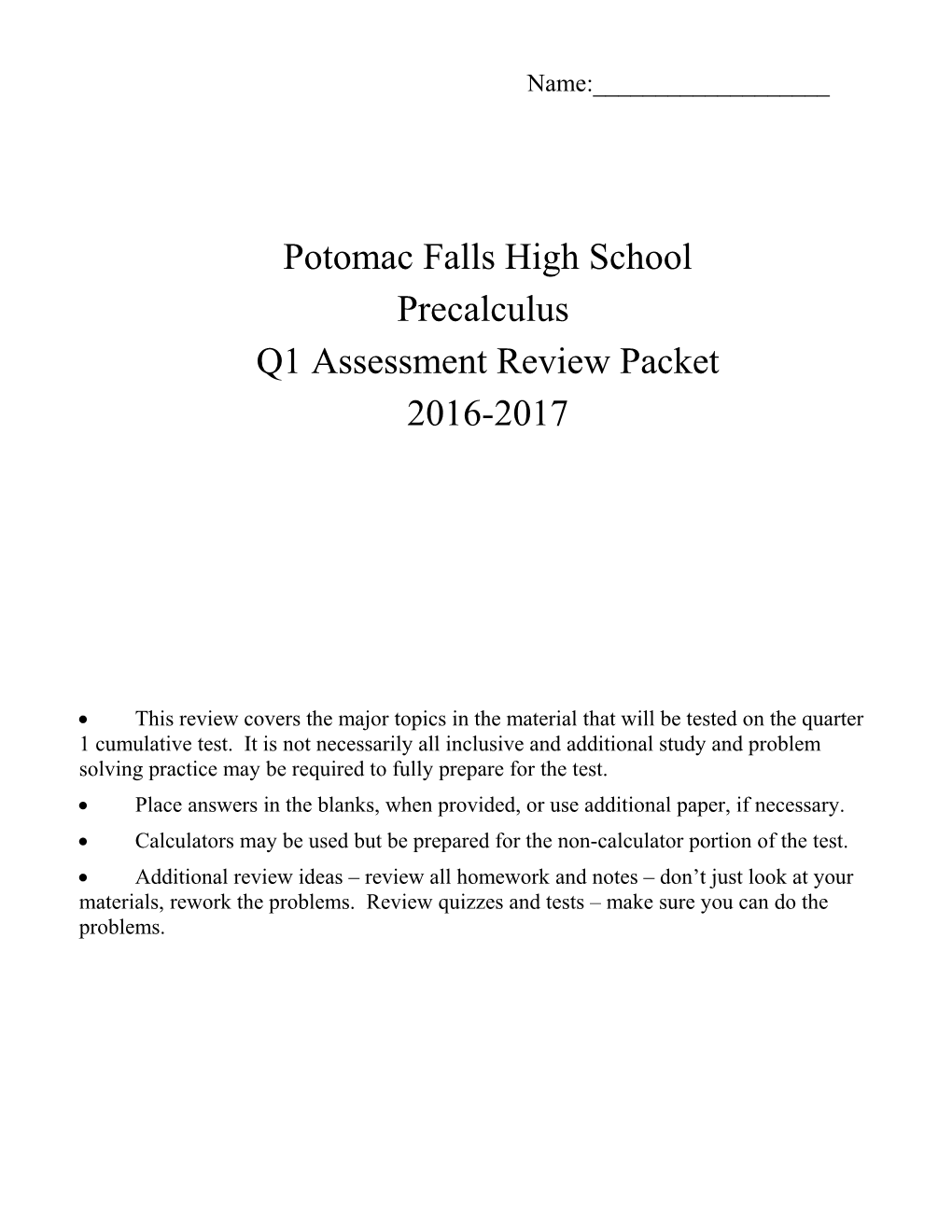# Geometry Semester Exam-Chapters 1-6Name:______

Potomac Falls High School

Precalculus

Q1 Assessment Review Packet

2016-2017

• This review covers the major topics in the material that will be tested on the quarter 1 cumulative test. It is not necessarily all inclusive and additional study and problem solving practice may be required to fully prepare for the test.
• Place answers in the blanks, when provided, or use additional paper, if necessary.
• Calculators may be used but be prepared for the non-calculator portion of the test.
• Additional review ideas – review all homework and notes – don’t just look at your materials, rework the problems. Review quizzes and tests – make sure you can do the problems.

FUNCTIONS

1 Find the following given

a) b) c)

2) Find the domain and range of each function:

a) D: ______
R: ______/ b) D: ______
R: ______

3) Find the inverse of each function. Is the function one-to-one?

a. b.

4) Graph each function and determine its domain and range.

a)
D: ______
R: ______/ b)
D: ______
R: ______

5) Determine whether each function is even, odd, or neither. Show your algebraic check.

a) / b)

6) Graph the inverse of the following graphs:.

a) / b)

7) Evaluate the following function:

8) For the graph below, state the intervals(in interval notation) for which the function is increasing or decreasing. Also what are the values of any local minima or maxima in this graph?

a) Increasing:

b) Decreasing:

c) Local min(s):

d) Local max(s):

9) Factor completely:

a) 2x2 + 15x + 7b) 3x2 – 5x – 12c) 9x2 + 11x + 2d) 7x2 – 22x + 3

d) y2 – 6y + 8e) 64x2 – 81f)

10) Write the following equation in vertex form: – you must use completing the square:

11) Top of Form
Which graph is not a function?
a) b) c) d)
Bottom of Form

12) Using the graph of as a guide, describe the transformations and then graph the function:

MATRICES

State the dimensions of the following matrices. Solve for the variable(s):

1. 2. 3.

Find the product. If the product is not defined, explain why.

4. 5. 6.(Calculator active)

Write an inventory matrix, a cost per item matrix and then determine a total cost matrix.

7. A softball team needs to buy 12 bats at \$21 each, 45 balls at \$4 each, and 15 uniforms at \$30 each.

8. A teacher is buying supplies for two art classes. For class 1, the teacher buys 24 tubes of paint, 12 brushes, and 17 canvasses. For class 2, the teacher buys 20 tubes of paint, 14 brushes, and 15 canvasses. Each tube of paint costs \$3.35, each brush costs \$1.75, and each canvass costs \$4.50.(Calculator active)

Questions 9 - 10: Find the inverse of each matrix, if it exists. No calculator.

9. 10.

Questions 11 - 12: Solve for X. 2x2 by hand, 3x3 with calculator.

11. 12. (Calculator active)

Questions 13-14: Solve the following systems using matrices. Check your answers. All 2x2 systems

must be solved by hand. Systems 3x3 and larger may be solved with a calculator.

However, show the matrix equation for all systems.

13. 14.

15. The vertices of a triangle are (–2, 2), (1, 5), and (6, -1). Find the area of the triangle using matrices. Round your answer to 2 decimal places in square units.

16. Find the function for which and using matrices.

17. The following matrix was used to encode the message into the number string you received: Message: 156 57 53 207 166 204 112 5 0 35 -32 -57 117 29 62 181 121 139

Your mission is to find the inverse of the encoding matrix, place the received message into an appropriate matrix, decrypt the message you received and translate back to an English sentence.

A / B / C / D / E / F / G / H / I / J / K / L / M
1 / 2 / 3 / 4 / 5 / 6 / 7 / 8 / 9 / 10 / 11 / 12 / 13
N / O / P / Q / R / S / T / U / V / W / X / Y / Z
14 / 15 / 16 / 17 / 18 / 19 / 20 / 21 / 22 / 23 / 24 / 25 / 26

Alphabet Code (space = 0)

Message: